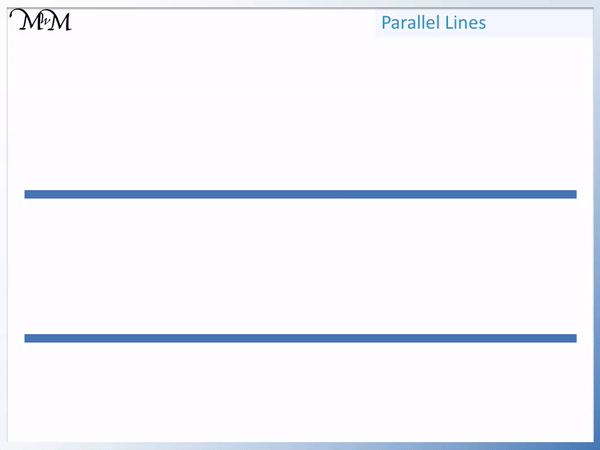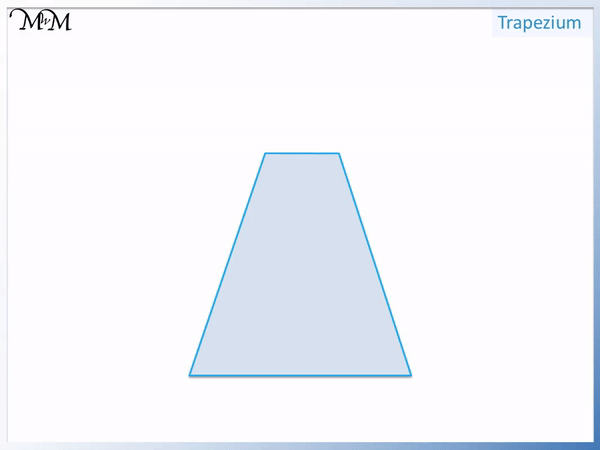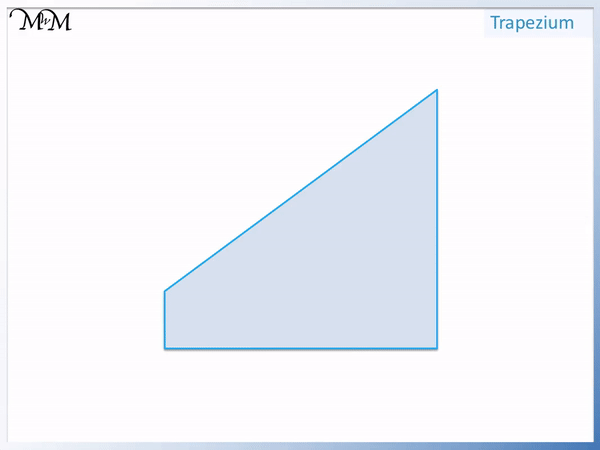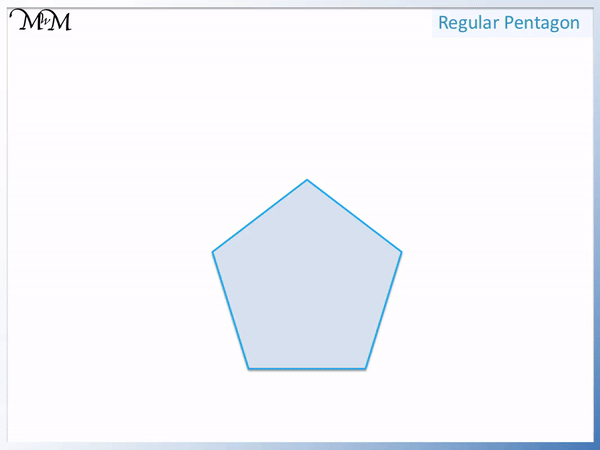# Parallel Sides

Parallel Sides• Parallel lines go in exactly the same direction.
• Two parallel lines never cross.
• Two parallel lines are always the same distance apart from each other.
• Parallel sides are two or more sides on a shape that are parallel.
• We mark a set of parallel sides on a shape with an arrow on each side.
• We mark further sets of parallel sides with two or more arrows if we have already marked a set with one arrow each.

Parallel sides go in exactly the same direction and do not touch.

Parallel sides are always the same distance apart.• Parallel sides are sides that do not cross.
• To check if sides are parallel, extend the sides with a ruler to see if they cross.
• If the sides cross, they are not parallel.
• We have one pair of parallel sides marked with a single red arrow.
• We have a second pair of parallel sides marked with two green arrows.
• This shape is a parallelogram.
• Parallelograms are shapes that have two pairs of parallel sides.Supporting Lessons# Shapes with Parallel Sides

## What are Parallel Lines?

Parallel lines are lines that do not cross. Parallel lines go in the same direction and remain the same distance apart. Therefore parallel lines do not cross.Parallel lines can be thought of as being like the two rails on train tracks. They always remain the same distance apart and head in the same direction.Parallel sides on shapes will almost always be straight sides, however it is possible to have curved parallel lines.

## How do you Show Parallel Lines on a Shape?

Some shapes will have sides that go in exactly the same direction and are therefore parallel.

The symbol for parallel lines is an arrow. An arrow is drawn on every side that is parallel to another. It does not matter which direction each arrow faces as long as it is in the same direction as the arrow on the side that it is parallel to.

Usually one arrow is drawn on each side that is parallel. However if the shape has another set of parallel sides, then two arrows are drawn on these sides. If there is another set of parallel sides, then three arrows would be drawn etc.

For example, the square below has two pairs of parallel sides.

The first pair of parallel sides shown are the two horizontal sides. Horizontal sides go from left to right, in the same direction as the horizon.

We mark this first pair of parallel sides with a single arrow.The second pair of parallel sides on this square are the two vertical sides. Vertical sides go from down to up.

We mark this next set of parallel sides with two arrows.

The sides with one arrow on are parallel to each other and the sides with two arrows on are parallel to each other.

The sides with one arrow on are not parallel to the sides with two arrows on.

When writing, we can use the symbol ∥ to mean parallel.

∥ is the symbol which means parallel to.

## How to Find Parallel Lines on a Shape

To find parallel lines on a shape, extend each side of the shape with a ruler. If the lines cross, then the lines are not parallel. The lines are parallel if they do not cross.

Alternatively, a ruler can be placed in line with one of the sides. When moved to another side without rotation, the ruler will be in line with any other parallel sides.

Horizontal and vertical lines are the easiest parallel lines to spot. These sides will be parallel to the edges of any paper or screens that they are displayed on.

It is also helpful to be aware of common shapes that do and do not have parallel lines. We saw above that a square has two pairs of parallel sides. A square is a special type of rectangle which has all sides the same length.

Below is a rectangle.

A rectangle has two pairs of parallel sides.

One pair of parallel sides is horizontal and the other pair of parallel sides are vertical.We label the first set of parallel sides with one arrow and the second pair of parallel sides with two arrows.

Below is a isosceles trapezium. Isosceles trapeziums are symmetric.

We can look for parallel sides by extending the sides with a ruler.

Remember to look for horizontal and vertical sides.

We can see that there is a pair of parallel sides that both go horizontally from left to right.We can extend the other two sides to check if they are parallel.

We can see that these other two sides cross. Parallel lines do not cross and therefore, these two sides are not parallel.

Here is a different trapezium.

We first check any horizontal and vertical sides to see if they are parallel.

We have a pair of vertical lines going from down to up.

Because they are both vertical, they are both parallel. We mark these parallel lines with an arrow.We can see that the other two sides cross when extended and so, they are not parallel sides.

A trapezoid always has one pair of parallel sides.

The shape below is called a parallelogram. It has the word parallel in its name.

We can see that it has two horizontal sides, which are parallel.

It also has another pair of parallel sides, which we mark with two arrows.A parallelogram has two pairs of parallel sides. Each side is always parallel to the side that is opposite to it.

A parallelogram is any four-sided shape that contains two pairs of parallel sides, where each side is parallel to the one opposite.

Therefore a rectangle, square and rhombus are all special kinds of parallelogram.

Many shapes do not have any parallel sides.

The shape in the example below is a regular pentagon, which has 5 sides that are all of the same length.

To check if there are any parallel lines on the sides of this shape, we can extend them with a ruler. If two lines cross then they are not parallel and they are parallel if they do not cross.

We can see that no matter which two sides we take, extending the lines will always result in the lines crossing.

No sides of this shape are parallel.Regular pentagons do not have any parallel sides.

Triangles are shapes that have no parallel sides. All triangles will have three sides and it is impossible for any of them to be parallel.

If two lines out of three were parallel, it would be impossible to draw the shape. You can start by drawing any two parallel lines and then trying to complete the shape. It is impossible to do by only using three sides in total.

All types of triangle, such as equilateral triangle, isosceles triangle and scalene triangle, have no parallel lines..

A kite is another shape that does not have parallel sides.

Some shapes have many parallel sides.

For example, a regular hexagon has three pairs of parallel sides. Each side is parallel to the side that is opposite to it.

A regular octagon is a shape that has 4 pairs of parallel sides. Each side is parallel to the side that is opposite to it.Now try our lesson on Sorting 2D Shapes where we learn how to sort shapes by their properties.error: Content is protected !!